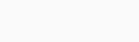# Solved – How to Calculate a Simple Case Kernel Density

My understanding of kernel densities is more intuitive than it is formal. I get the whole idea that observations that are far away from Xi are down-weighted, resulting in smoothing. However, the vast majority of tutorials on the subject use built-in code for this, and I'm a little lost when looking at using the formula to compute this from scratch.

There are many different smoothing formulas. Lets just use one of the most common:I understand the first part only: three fourths times (one minus resid squared), but what does the latter inequality mean? is that the domain? or is it i as in imaginary number? I don't find it particularly clear.

If someone could provide a short worked example of computing the first few values of a kernel density series, that would be ideal.

Contents

You are asking about two things: kernel density estimation and some particular kernel used in kernel density estimation. For the first question you can find some introduction in Can you explain Parzen window (kernel) density estimation in layman's terms? and How to interpret the bandwidth value in a kernel density estimation? threads.

\$\$ frac{3}{4} (1 – u^2) ; I(|u| le 1) \$\$

it is called Epanechnikov kernel (called after Epanechnikov, 1969). The equation can be split into three parts,

1. the normalizing constant \$frac{3}{4}\$ that makes it integrate to unity, so that it is a proper probability distribution;
2. the main body of the kernel function \$(1 – u^2)\$;
3. and the indicator function \$I(|u| le 1)\$ that makes it zero everywhere outside the \$[-1, 1]\$ domain.

There is not much to say about it, it simply returns probability density proportional to one minus squared distance from it's center. This makes it related to mean squared error and optimal in terms of it. Nonetheless, this does not make much difference in practice as the choice of kernel is not of great importance in kernel density estimation.

If you are in doubt what the function does, you can always plot it to gain more intuition:Epanechnikov, V.A. (1969). Non-parametric estimation of a multivariate probability density. Theory of Probability and its Applications. 14: 153–158.

Rate this post